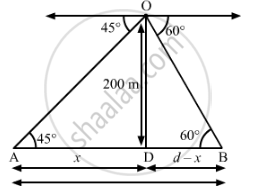# Two ships are there in the sea on either side of a light house in such a way that the ships and the light house are in the same straight line. The angles of depression of two ships as observed from the top of the light house are 60° and 45°. If the height of the light house is 200 m, find the distance between the two ships. [use √3=1.73] - Mathematics

Two ships are there in the sea on either side of a light house in such a way that the ships and the light house are in the same straight line. The angles of depression of two ships as observed from the top of the light house are 60° and 45°. If the height of the light house is 200 m, find the distance between the two ships. [use √3=1.73]

#### Solution

Let d be the distance between the two ships. Suppose the distance of one of the ships from the light house is x metres, then the distance of the other ship from the light house is (dx) metres.In right-angled ∆ ADO, we have:

tan 45^@="OD"/"AD"=200/x

=>1=200/x

=>x=200  ............(1)

In right-angled ∆ BDO, we have:

tan60^@="OD"/"BD"=200/(d-x)

=>sqrt3=200/(d-x)

=>d-x=200/sqrt3

Putting x= 200, we have:

d-200=200/sqrt3

d=200/sqrt3+200

=>d=200((sqrt3+1)/sqrt3)

=>d=200xx1.58

=>d=316   (approx)

Thus, the distance between two ships is approximately 316 m

Concept: Heights and Distances
Is there an error in this question or solution?